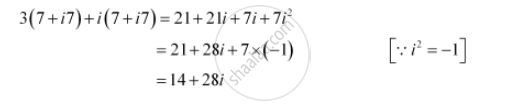CBSE (Arts) Class 11CBSE
Share

# Express the Given Complex Number in the Form A + Ib: 3(7 + I7) + I(7 + I7) - CBSE (Arts) Class 11 - Mathematics

#### Question

Express the given complex number in the form a + ib: 3(7 + i7) + i(7 + i7)

#### SolutionIs there an error in this question or solution?

#### Video TutorialsVIEW ALL 

Solution Express the Given Complex Number in the Form A + Ib: 3(7 + I7) + I(7 + I7) Concept: Complex Numbers.
S